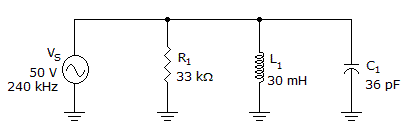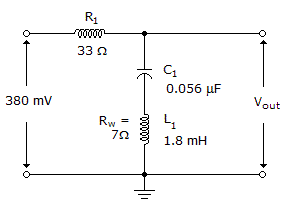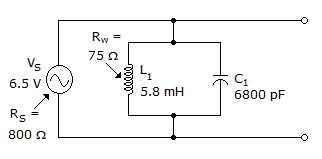# Electronics - RLC Circuits and Resonance

### Exercise :: RLC Circuits and Resonance - Filling the Blanks

1.

When a parallel tank is used in series with an output load resistor the filter is a(n) ______ filter.

 A. band-stop B. low-pass C. bandpass D. high-pass

Answer: Option A

Explanation:

No answer description available for this question. Let us discuss.

2.The phase angle is ______ in the given circuit.

 A. 34.5° B. 43.3° C. 46.7° D. 55.5°

Answer: Option C

Explanation:

No answer description available for this question. Let us discuss.

3.

The ___ of a circuit describes the ability of that circuit to respond to certain frequencies while rejecting all others.

 A. bandwidth B. selectivity C. sensitivity D. quality factor

Answer: Option B

Explanation:

No answer description available for this question. Let us discuss.

4.The output voltage is _____ at the resonant frequency in the given circuit.

 A. 0 mV B. 31.4 mV C. 66.5 mV D. 380 mV

Answer: Option C

Explanation:

No answer description available for this question. Let us discuss.

5.The output voltage at the resonant frequency is ______ in the given circuit. If a 10 kload is connected.

 A. 553 mV B. 5.65 V C. 6.01 V D. 6.5 V

Answer: Option B

Explanation:

No answer description available for this question. Let us discuss.

#### Current Affairs 2021

Interview Questions and Answers